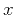Asymptotic pseudounitary stacking operatorsNext: Migration Up: EXAMPLES Previous: EXAMPLES

## Datuming

Letdenote a point on the surface at which the propagating wavefield is recorded. Letdenote a point on another surface, to which the wavefield is propagating. Then the summation path of the stacking operator for the forward wavefield continuation is(32)

whereis the time recorded at the-surface, andis the traveltime along the ray connectingand. The backward propagation reverses the sign in (32), as follows:(33)

Substituting the summation path formulas (32) and (33) into the general weighting function formulas (28) and (29), we immediately obtain(34)

Gritsenko's formula (Goldin, 1986; Gritsenko, 1984) states that the second mixed traveltime derivativeis connected with the geometric spreadingalong the-ray by the equality(35)

whereis the velocity at the point, andandare the angles formed by the ray with theandsurfaces, respectively. In a constant-velocity medium,(36)

Gritsenko's formula (35) allows us to rewrite equation (34) in the form (Goldin, 1988)(37)(38)

The weighting functions commonly used in Kirchhoff datuming (Wiggins, 1984; Berryhill, 1979; Goldin, 1985) are defined as(39)(40)

These two operators appear to be asymptotically inverse according to formula (10). They coincide with the asymptotic pseudo-unitary operators if the velocityis constant (), and the two datum surfaces are parallel ().Asymptotic pseudounitary stacking operatorsNext: Migration Up: EXAMPLES Previous: EXAMPLES

2013-03-03